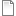# Model Checking Games for the Alternation Free mu-Calculus and Alternating Automata

 Title Model Checking Games for the Alternation Free mu-Calculus and Alternating Automata Publication Type Conference Paper Year of Publication 1999 Authors Leucker, M Editor Ganzinger, H, McAllester, D, Voronkov, A Conference Name Proceedings of the 6th International Conference on Logic for Programming and Automated Reasoning "(LPAR'99)" Series Lecture Notes in Artificial Intelligence Volume 1705 Publisher Springer Abstract We relate game-based model checking and model checking via {\em 1-letter simple weak alternating {B}üchi automata} (1SWABA) for the {\em altern\-ation-free $μ$-calculus}. Game-based algorithms have the advantage that in addition to checking whether a formula is valid or not they determine a winning strategy which can be employed for explaining to the user why the formula is valid or not. 1SWABA are a restricted class of alternating {B}üchi automata and were defined in \cite{BVW94}. They admit efficient automata-based model checking for CTL and the alternation-free $μ$-calculus. We give an interpretation for these automata in terms of game theory and show that this interpretation coincides with the notion of model checking games for CTL and the $μ$-calculus. Then we explain that the efficient non-emptiness procedure for 1SWABA presented in \cite{BVW94} can also be understood as a game-based model checking procedure. Furthermore, we show that this algorithm is not only useful for checking the validity of a formula but also for determining a {\em winning strategy} for the winner of the underlying model checking game. In this way we obtain a linear time algorithm for model checking games. URL http://www-i2.informatik.rwth-aachen.de/leucker/Literatur/Ps_files/leucker__Games_Automata_mu_calculus.ps.gz
Bibtex:
@inproceedings {L99,
title = {Model Checking Games for the Alternation Free mu-Calculus and Alternating Automata},
booktitle = {Proceedings of the 6th International Conference on Logic for Programming and Automated Reasoning "(LPAR{\textquoteright}99)"},
series = {Lecture Notes in Artificial Intelligence},
volume = {1705},
year = {1999},
pages = {77{\textendash}91},
publisher = {Springer},
organization = {Springer},
abstract = {We relate game-based model checking and model checking via {\em 1-letter simple weak alternating {B}{\"u}chi automata} (1SWABA) for the {\em altern\-ation-free $μ$-calculus}. Game-based algorithms have the advantage that in addition to checking whether a formula is valid or not they determine a winning strategy which can be employed for explaining to the user why the formula is valid or not. 1SWABA are a restricted class of alternating {B}{\"u}chi automata and were defined in \cite{BVW94}. They admit efficient automata-based model checking for CTL and the alternation-free $μ$-calculus. We give an interpretation for these automata in terms of game theory and show that this interpretation coincides with the notion of model checking games for CTL and the $μ$-calculus. Then we explain that the efficient non-emptiness procedure for 1SWABA presented in \cite{BVW94} can also be understood as a game-based model checking procedure. Furthermore, we show that this algorithm is not only useful for checking the validity of a formula but also for determining a {\em winning strategy} for the winner of the underlying model checking game. In this way we obtain a linear time algorithm for model checking games.},
url = {http://www-i2.informatik.rwth-aachen.de/leucker/Literatur/Ps_files/leucker__Games_Automata_mu_calculus.ps.gz},
author = {Martin Leucker},
editor = {Harald Ganzinger and David McAllester and Andrei Voronkov}
}
Postscript:games_automata_mu_calculus.ps_1.gz